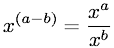Equations > Algebra > Exponents/Powers > Subtraction of Exponents Rule

### Subtraction of Exponents RuleLatex Code:

MathML Code:

 ${x}^{\left(a-b\right)}=\frac{x}^{a}{x}^{b}$

MathType 5.0: